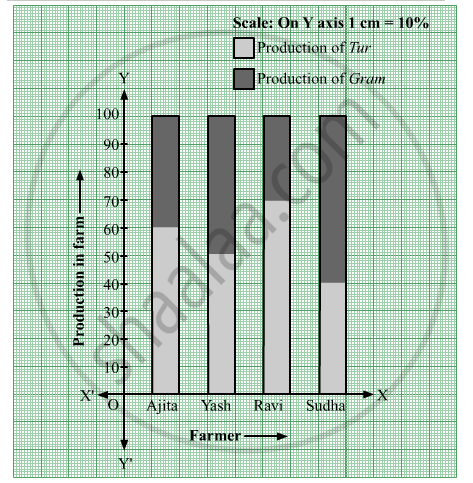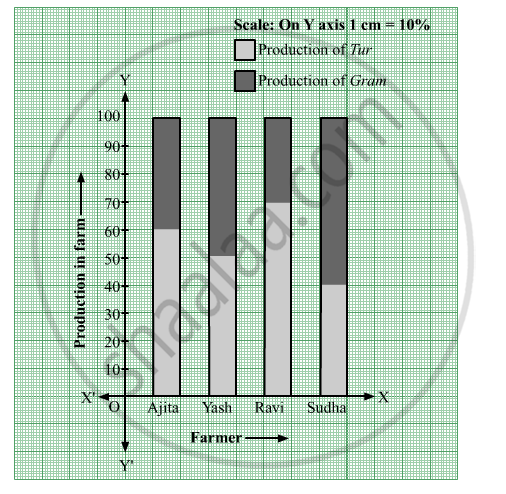SSC (English Medium) Class 8Maharashtra State Board
Share

# Observe the Following Graph and Answer the Questions. - SSC (English Medium) Class 8 - Mathematics

ConceptPercentage Bar Graph/Diagram

#### Question

Observe the following graph and answer the questions.(1) State the type of the bar graph.
(2) How much percent is the Tur production to total production in Ajita'a farm?
(3) Compare the production of Gram in the farms of Yash and Ravi and state whose percentage of production is more and by how much?
(4) Whose percentage production fo Tur is the least?
(5) State production percentages of Tur and gram in Sudha's farm

#### Solution(1) This is a percentage bar graph.

(2) The Tur production is 60% of the total production in Ajita'a farm.

(3) The production of gram in the farm of Yash is 50%, whereas

The production of gram in the farm of Ravi is 30%.

Since, 50 - 30 = 20

So, the production of Gram in the farms of Yash is more than that of Yash by 20%.

(4) The production fo Tur in the farm of Sudha is the least, i.e. 40%.

(5) The production percentages of Tur and gram in Sudha's farm are 40% and 60%, repectively.

Is there an error in this question or solution?

#### APPEARS IN

Balbharati Solution for Balbharati Class 8 Mathematics (2019 to Current)
Chapter 11: Statistics
Practice Set 11.3 | Q: 2 | Page no. 74
Solution Observe the Following Graph and Answer the Questions. Concept: Percentage Bar Graph/Diagram.
S